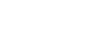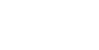# Calculation of acoustic bass reflex online

Created on 03/15/2011 01:34 AM.

Updated 09.20.

Author: Yu. Lyubimov, P. Sayk, E. Vinogradova, M. Ephrussi.

The proposed methods for calculating the phase inverter are based on the simplest measurements carried out with a well-defined specimen of the loudspeaker installed in the acoustic phase inverter and on the nomographic determination of the dimensions of the latter. As well as options for calculating the phase inverter using the technical parameters of the speakers from the manufacturer.

## What is a Bass Reflex?

The phase inverter is a pneumo-acoustic Helmholtz resonator.

And like any resonator, it has a very pronounced resonance, with a high Q factor and steep slopes. So, in the case of the sub, we have two resonators: active (speaker) and passive (phase inverter). Both of them influence each other through an elastic medium (air).

The purpose of the FI is to reduce the lower operating frequency of the subwoofer, to add low frequencies.

## What should be the resonance frequency of the bass reflex?

The resonance frequency of the bass reflex (in the general case) should be 1/3 (33%) lower than the resonance frequency of the same speaker in the same box with the phase inverter opening closed.

Example:
Fres. dynamics in ZA = 60 Hz.
60 x 0.33 = 20Hz.
60-20 = 40 Hz.

The calculated resonant frequency of the FI in this example should be 40 Hz.

For this frequency, and I consider further the diameter and length of the phase inverter for the volume of this box. Now let’s look at more scientific options for calculating the phase inverter.

## Option number 1. A simple option for calculating the dimensions of the bass reflex

This option is suitable for the lazy. We need to know the frequency of tuning the bass reflex for this speaker. It is often indicated by speaker manufacturers in specifications such as packaging.where:

• F is the frequency of adjusting the FI;
• C is the speed of sound;
• n – number = 3.14 …;
• S is the area of ​​the hole;
• L – effective pipe length (pipe length plus 5 percent);
• V is the volume of the body.

Everywhere meters and hertz.

Accordingly, the ratio of the hole area to the length of the bass reflex:that is, when the hole area doubles (two ports), the length of each phase inverter doubles. It is impractical to make a narrow bass reflex to reduce the length of the pipe – the flow rate in it increases (there should be no more than 5% of the speed of sound! I was mistaken from memory)

It also makes no sense to make a very wide and long phase inverter at the same time – its length should not be more than the wavelength at the resonance frequency, so that there are no standing waves, but in fact it turns out several meters, so it is difficult to make a mistake here.

## Option number 2

First of all, guided by Fig. 1 and the table, it is necessary to make a “standard volume” – a sealed plywood box, all joints of which, in order to avoid air leaks, are carefully fitted, glued and coated with plasticine.

Diffuser diameter
loudspeaker, mm
Dimensions, mm
A V WITH
200 255 220 170
250 360 220 220
300 360 220 270
375 510 220 335

Next, measure the natural frequency of the resonance of the loudspeaker in free space. To do this, it is suspended in the air away from large objects (furniture, walls, ceiling). The measurement scheme is shown in Fig. 2.

Here ZG is a graduated sound generator, V is an alternating current tube voltmeter and R is a 100–1000 ohm resistor (at high resistance values, the measurement turns out to be more accurate).

Rotating the frequency adjustment knob of the sound generator in the range from 15-20 to 200-250 Hz, achieve the maximum deflection of the voltmeter needle. The frequency at which the deflection is maximum and is the resonant frequency of the loudspeaker in free space Fv

The next step is to determine the resonant frequency of loudspeaker Fv when it works at the “standard volume”. To do this, place the loudspeaker on the “standard volume” opening with a diffuser and press it slightly in order to avoid air leaks at the junction of the surfaces. The method for determining the resonance frequency is the same, but in this case it will be 2–4 times higher.

Knowing these two frequencies, the dimensions of the phase inverter are found with the help of nomograms. Depending on the diameter of the loudspeaker diffuser, select the nomogram shown in Fig. 3 (for diameter 200 mm), in fig. 4 (for diameters 250 and 300 mm) or fig. 5 (for a diameter of 375 mm). According to the selected nomogram, the volume of the phase inverter is determined, for which the points corresponding to the found frequencies are connected with a straight line on the axes “Resonant frequency”

Fv (see Fig. 4 point A) and “Resonant frequency” FI am (point B). The point of intersection with the auxiliary axis is marked, and from here a second straight line is drawn through point D to the “optimal volume” axis. The value corresponding to the new intersection point E is the desired volume.

If there are no special considerations for designing a box of a special configuration, then the calculation of its internal dimensions for a given volume can be done according to the nomogram shown in Fig. 6. The width of the bass reflex will be 1.4 times the height and the height 1.4 times the depth. The use of the nomogram is not difficult: a straight line is drawn between the extreme axes on which the volume values ​​are plotted. The intersection points of the straight line with the axes A, B, C will determine the width, height and depth of the box. The diameter of the loudspeaker cutout is taken equal to the C dimension shown in the table.

Further, having asked the diameter of the tunnel, it is necessary to determine its length and check whether it fits into the phase inverter box. The length of the tunnel is found from the graphs shown in Fig. 7, for three internal diameters: graphs A – for a diameter of 50 mm, B – for a diameter of 75 mm and B – for a diameter of 120 mm. Having chosen the appropriate graphs, by frequency Fv and the volume of the phase inverter, determined earlier, find the length of the tunnel (example in Fig. 7, B). It should be 35–40 mm less than the inner depth of the box. If this does not work, you can change the configuration of the box in any way, keeping its volume, or take a different diameter of the tunnel.